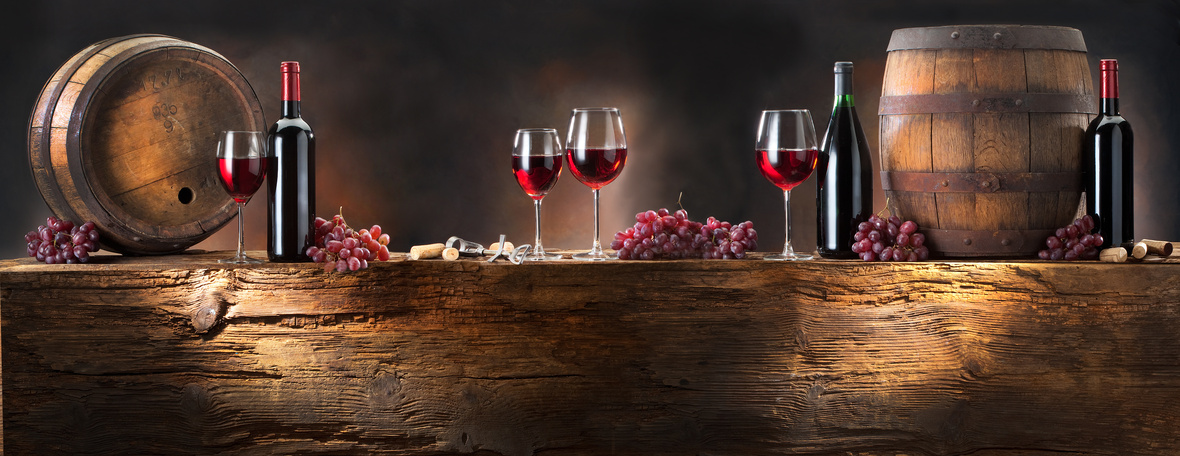# Holiday Gifting Energy and Giving Thanks

 /* styles */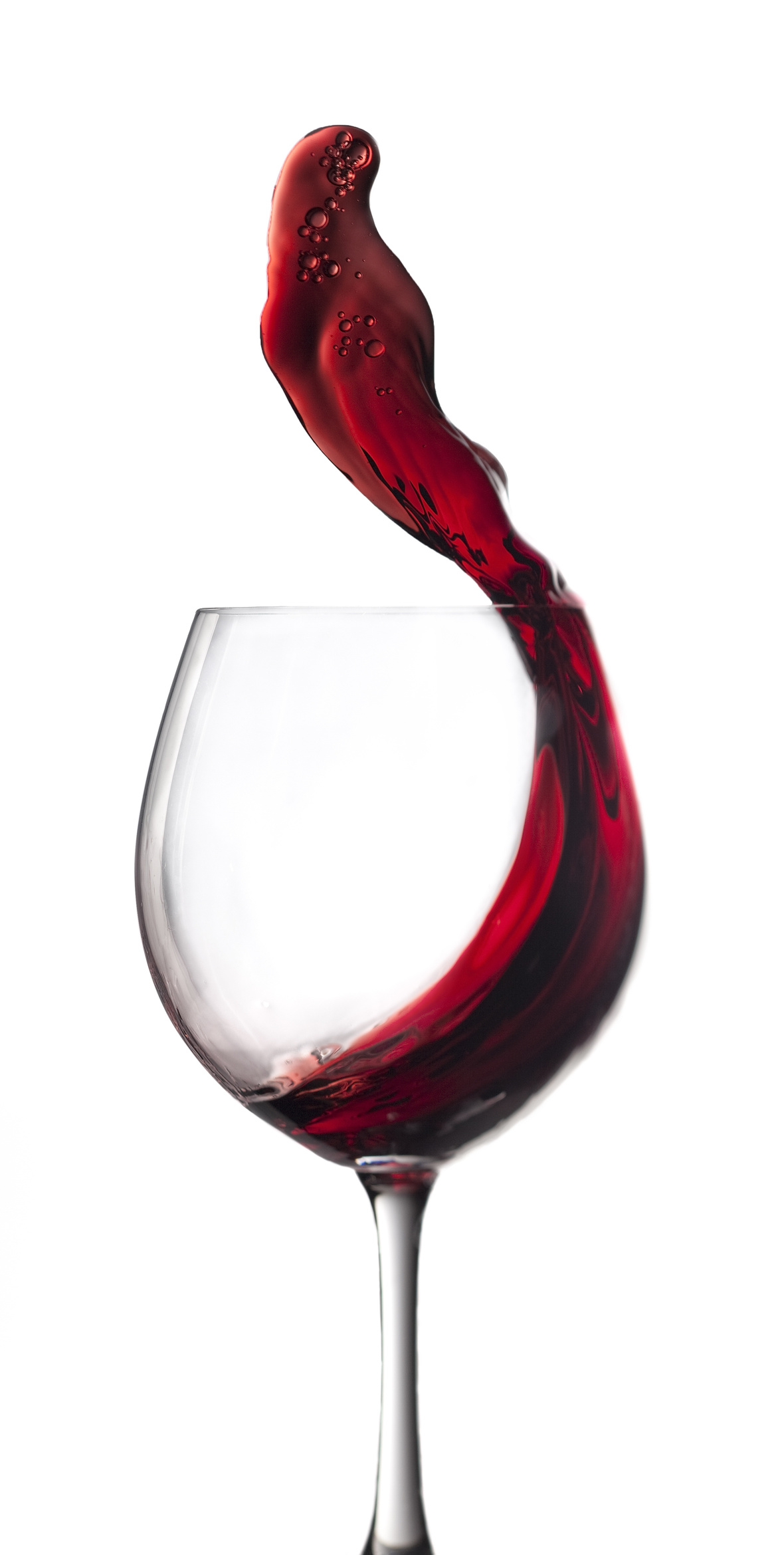Hi Wine & Spirits Lover,

The Shall We Wine team is excited to share our monthly newsletter. Our goal is to introduce you to our favorite selections, producers, distillers, varietals, regions and places to purchase and drink fabulous wine and spirits!

Cheers,

The Shall We Wine Team

 /* styles */

## GIVE THE GIFT OF THE SHALL WE WINE EXPERIENCE/* styles */Did you know we now offer gift cards? Spread some ★ holiday magic ★ and let your loved ones know they are appreciated (plus on Santa’s nice list) by gifting them a virtual stocking gift card from Shall We Wine! No fuss with physical plastic cards that you might misplace, this one is sent directly thru e-mail.

A gift that is sure to fit!

[ or ] bypass the guessing game and simply let your recipient choose how they want to spend their present. Fill any holiday stocking with these joys:

SWW Stocking Stuffer (\$15)
Can be used by recipient to join a cocktail hour or educational class

SWW Exclusive (\$500)
Can be used by recipient for an exclusive Private Virtual Event with Regine T. RousseauAdditional denominations of \$100, \$250, \$750 and \$1,000 are available as well! Fits all types of occasions, big or small. For redemption on Shall We Wine products and services.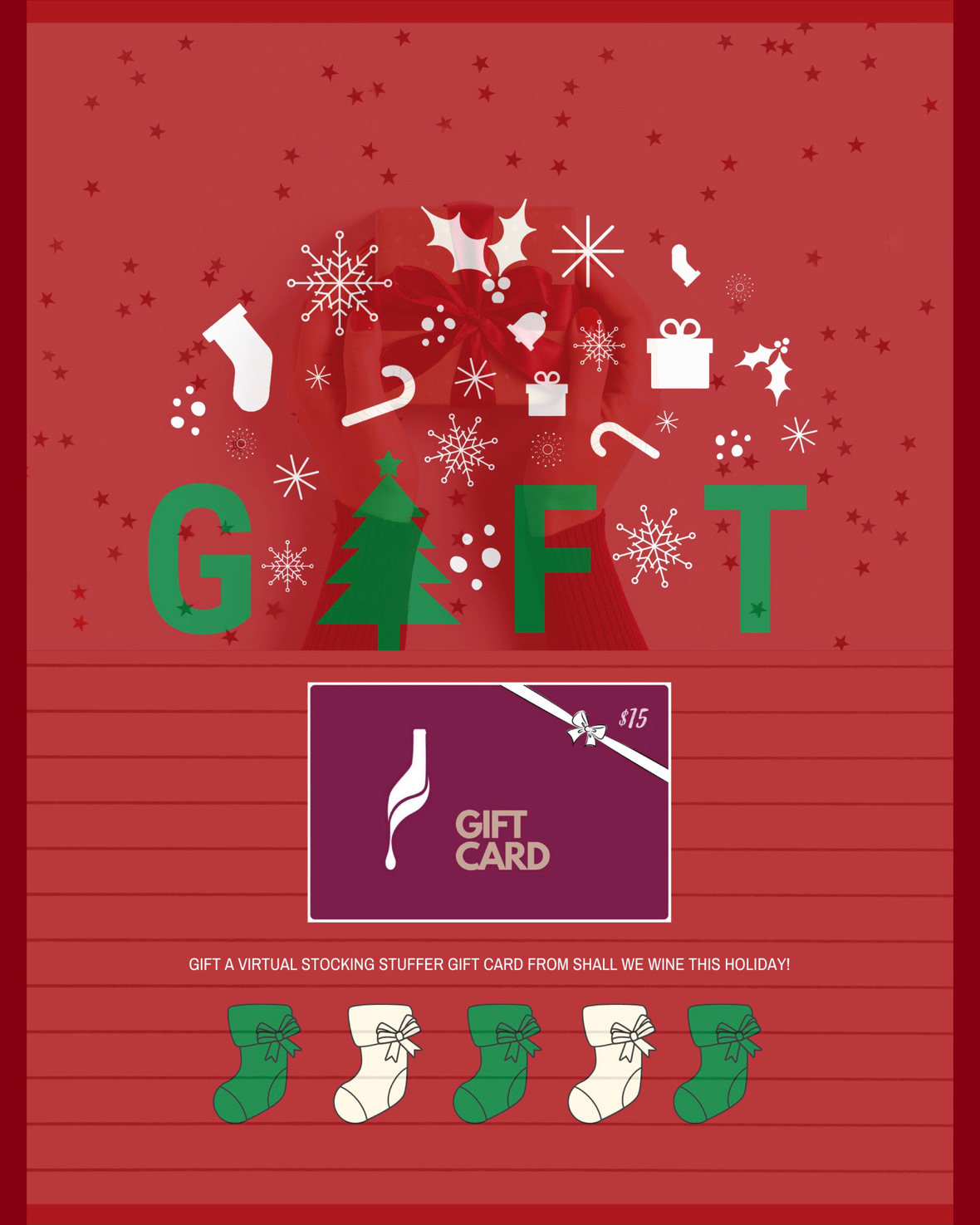table div table+table+table+table+table+table+table+table+table+table+table+table div table{width:100%;padding:0}table div table+table+table+table+table+table+table+table+table+table+table+table div table img{width:96.23%;padding:0;float:none}table div table+table+table+table+table+table+table+table+table+table+table+table div table td{width:100%;padding:0 1.88% 18px}/* styles */## SWW: THE MEMBERSHIPThe Ultimate Sip of Approval

What started as a gathering of wine and spirits lovers formed a community where members come to share, learn, and grow.

Our members are people who are interested in exploring wine, spirits, travel, books, art, health, wellness, advocacy and having deep and fun conversation around these interests.

We invite you to take a seat at our table.

 /* styles */ Shall We Wine Membership offers curated wine and spirits events led by industry. We offer a safe space to have thought-provoking conversations, and when the world allows us, we will take our members to our favorite destinations! Membership to Shall We Wine is our way of recognizing our most loyal wine tribe ⁠— the best way to access and take advantage of members-only perks. This is just the beginning.
 table div table+table+table+table+table+table+table+table+table+table+table+table+table+table+table+table div table{width:100%;padding:0}table div table+table+table+table+table+table+table+table+table+table+table+table+table+table+table+table div table img{width:96.23%;padding:0;float:none}table div table+table+table+table+table+table+table+table+table+table+table+table+table+table+table+table div table td{width:100%;padding:0 1.88% 18px}/* styles */## ANNOUNCING OUR 2021 WINE EDUCATION SERIES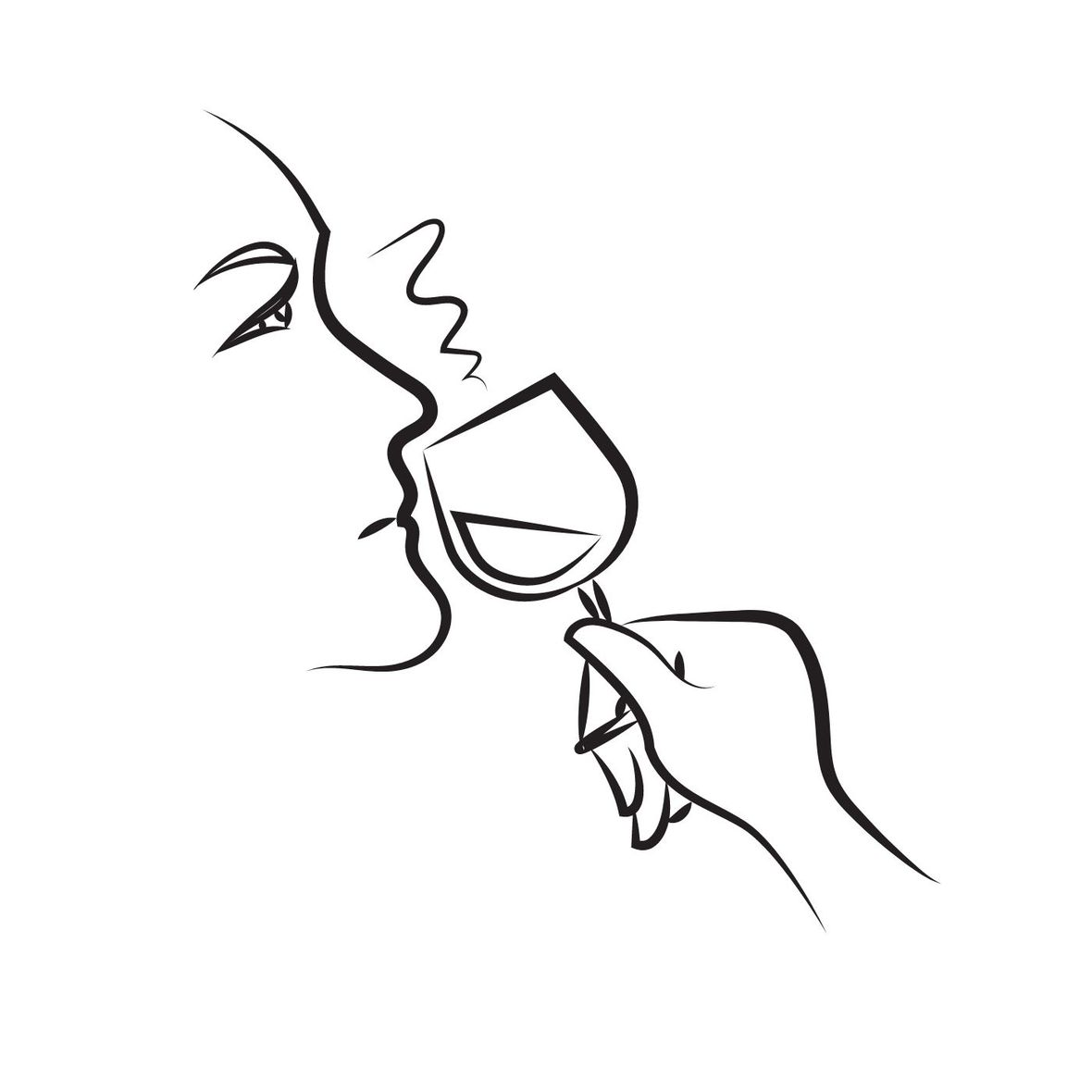We are excited to announce our wine education series for 2021! The SWW Fundamentals Class Series is designed for beginners to intermediate level wine lovers. The goal of these classes is to empower you to find the wines that fit your palate, the foods you enjoy and your mood.

If you enjoy wine and are looking to deepen your knowledge of wine styles, grapes and regions, this is the class for you!

Text Book - Wine Folly: The Essential Guide to Wine
(This book is FREE with a Premier Cru Membership)

 /* styles */ ★ Classes are FREE for Premier Cru members, \$40 per class for non members ★
 /* styles */January 2021
Six Elements of Wine Tasting

In this engaging workshop, I will share one of the most powerful wine tasting exercises I learned some years ago from Food and Wine Magazine. I will teach you how to identify the six elements of wine tasting; body, tannins, acidity, sweetness, flavors and oak. By the end of the workshop, you will clearly understand the six elements, how to identify them in wine and other foods, and be confident in communicating which of these aspects of these elements appeal to your palate!

February-December 2021
Find Your Wine Style - SWW Fundamentals Class Series

Our Fundamentals of Wine Series continues with a focus on helping you identify your wine style. Through clear and easy to understand infographics, we will learn about various wine styles, grapes varieties, regions and most importantly which is your personal favorite. Each Premier Cru Member will receive a copy for FREE of our textbook when signed up for the series.

SWW Fundamentals Class Series 2021 Schedule

February: Full Bodied Reds
March: Medium Bodied Red Wines
April: Light Bodied Reds and Rosé (Rosé, Rosado, Vin Gris)
May: Aromatic White Wines
June: Light- Bodied Whites Wines
July: No Class
August: No Class
September: Full Bodied White Wines
October: Dessert Wines
November: Sparkling Wines
December: No Class

 table div table+table+table+table+table+table+table+table+table+table+table+table+table+table+table+table+table+table+table+table+table+table div table{width:100%;padding:0}table div table+table+table+table+table+table+table+table+table+table+table+table+table+table+table+table+table+table+table+table+table+table div table img{width:96.23%;padding:0;float:none}table div table+table+table+table+table+table+table+table+table+table+table+table+table+table+table+table+table+table+table+table+table+table div table td{width:100%;padding:0 1.88% 18px}/* styles */## NEVER MISS A WINE & SPIRITS CONNECTION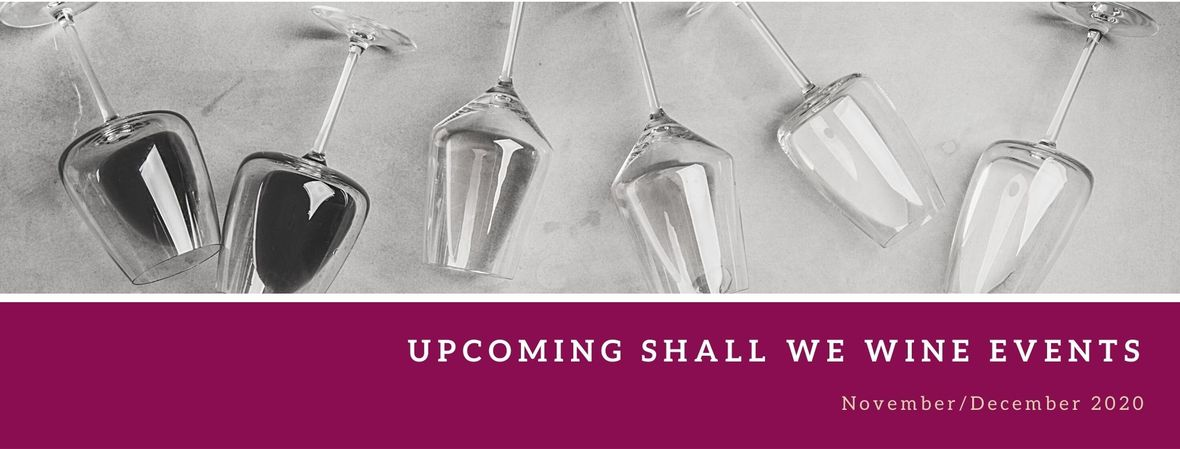/* styles */ Check out our newly updated events calendar to see what your next pour should be!table div table+table+table+table+table+table+table+table+table+table+table+table+table+table+table+table+table+table+table+table+table+table+table+table+table+table+table+table div table{width:100%;padding:0}table div table+table+table+table+table+table+table+table+table+table+table+table+table+table+table+table+table+table+table+table+table+table+table+table+table+table+table+table div table img{width:96.23%;padding:0;float:none}table div table+table+table+table+table+table+table+table+table+table+table+table+table+table+table+table+table+table+table+table+table+table+table+table+table+table+table+table div table td{width:100%;padding:0 1.88% 18px}/* styles */## DRINK THIS: THANKSGIVING WINE FLASHBACK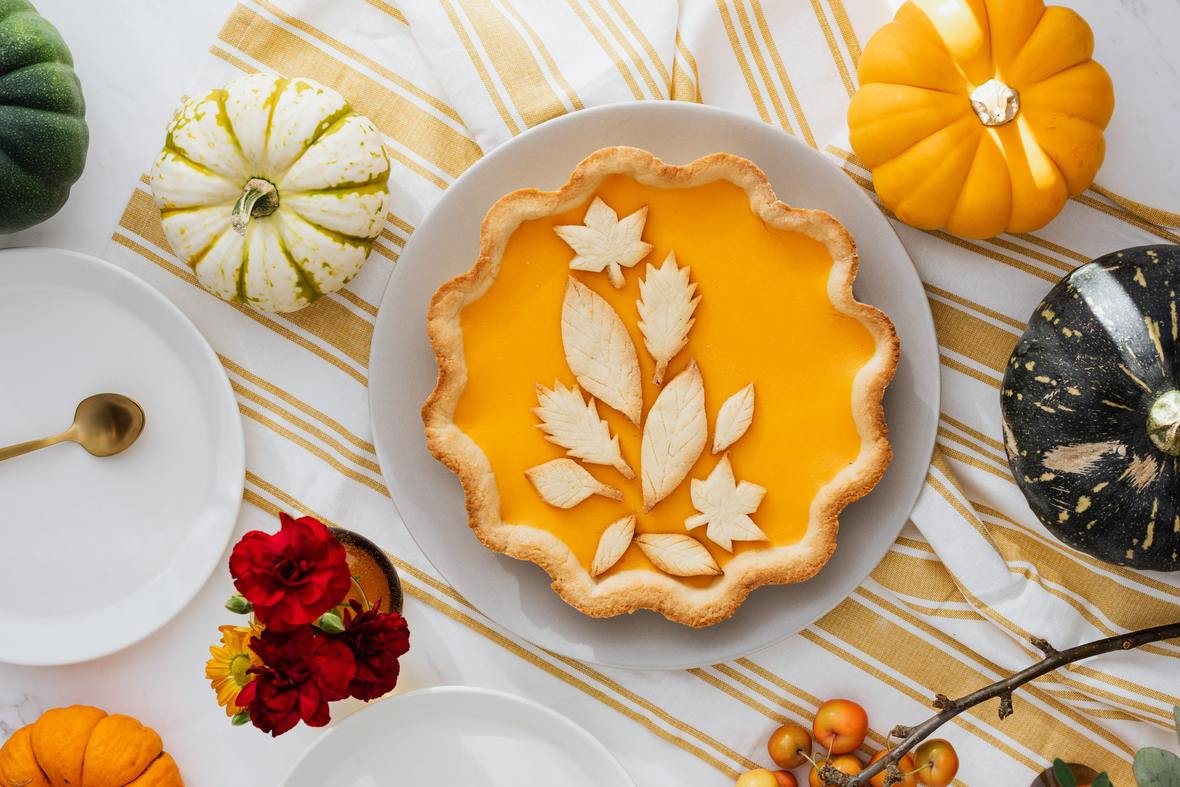Wine Pairings & Surviving the Holiday Dinner Commentary

Cheers to this Thanksgiving Pie flashback, because even if you're having the holiday dinner over a Zoom call, what might not change is the barrage of criticisms flowing from friends & family! That's perfectly ok. You still love them. You just need an assist... in this case, from dessert wine.

Go make a pie (or buy one), grab a bottle of wine before you turn your camera on and stay covered with this handy pie wine guide! From wine for those Backhanded Compliments to Awkward Silences or Small Talk, we've got you.

 table div table+table+table+table+table+table+table+table+table+table+table+table+table+table+table+table+table+table+table+table+table+table+table+table+table+table+table+table+table+table+table div table{width:100%;padding:0}table div table+table+table+table+table+table+table+table+table+table+table+table+table+table+table+table+table+table+table+table+table+table+table+table+table+table+table+table+table+table+table div table img{width:96.23%;padding:0;float:none}table div table+table+table+table+table+table+table+table+table+table+table+table+table+table+table+table+table+table+table+table+table+table+table+table+table+table+table+table+table+table+table div table td{width:100%;padding:0 1.88% 18px}/* styles */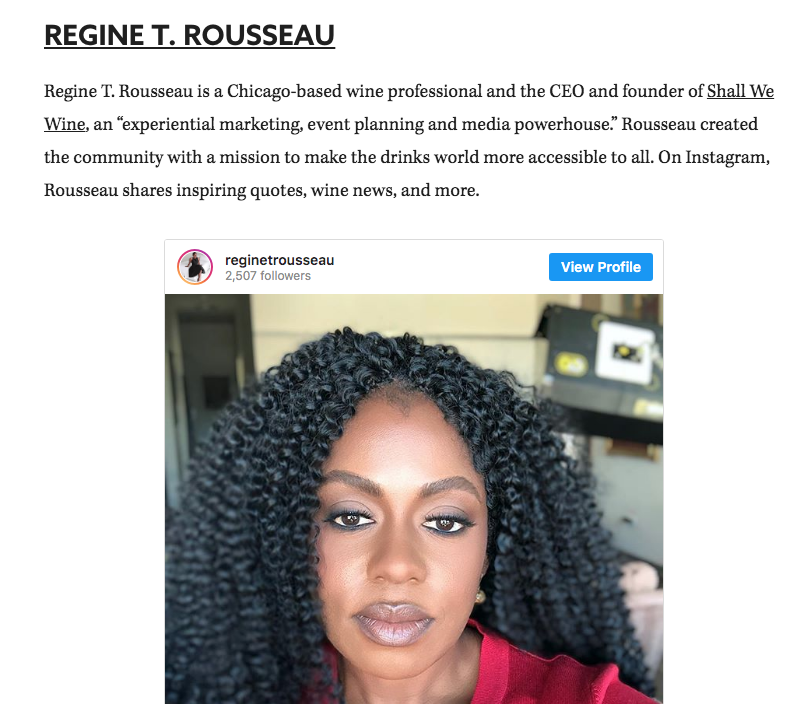15 Wine Experts You Should Be Following on Instagram

What a wonderful list.

Thank you, VinePair for the feature!

If you're looking to see cool wine enthusiasts to follow on social and don't know where to begin, this VinePair list is a fabulous starting point. Find out everything from what we're drinking and upcoming events not to be missed.

 /* styles */
 /* styles */ To stay in the know with the latest and greatest, make sure to follow us! Links are below./* styles */ CLICK BELOW
 table div table+table+table+table+table+table+table+table+table+table+table+table+table+table+table+table+table+table+table+table+table+table+table+table+table+table+table+table+table+table+table+table+table+table+table+table+table+table div table{width:100%;padding:0}table div table+table+table+table+table+table+table+table+table+table+table+table+table+table+table+table+table+table+table+table+table+table+table+table+table+table+table+table+table+table+table+table+table+table+table+table+table+table div table table{padding:0;float:left!important;width:26.059%!important}table div table+table+table+table+table+table+table+table+table+table+table+table+table+table+table+table+table+table+table+table+table+table+table+table+table+table+table+table+table+table+table+table+table+table+table+table+table+table div table td{padding-left:29px;padding-right:29px}table div table+table+table+table+table+table+table+table+table+table+table+table+table+table+table+table+table+table+table+table+table+table+table+table+table+table+table+table+table+table+table+table+table+table+table+table+table+table div table table+table,table div table+table+table+table+table+table+table+table+table+table+table+table+table+table+table+table+table+table+table+table+table+table+table+table+table+table+table+table+table+table+table+table+table+table+table+table+table+table div table table+table+table{float:left!important;width:26.059%!important}table div table+table+table+table+table+table+table+table+table+table+table+table+table+table+table+table+table+table+table+table+table+table+table+table+table+table+table+table+table+table+table+table+table+table+table+table+table+table div table table td,table div table+table+table+table+table+table+table+table+table+table+table+table+table+table+table+table+table+table+table+table+table+table+table+table+table+table+table+table+table+table+table+table+table+table+table+table+table+table div table table+table td,table div table+table+table+table+table+table+table+table+table+table+table+table+table+table+table+table+table+table+table+table+table+table+table+table+table+table+table+table+table+table+table+table+table+table+table+table+table+table div table table+table+table td{padding-left:0;padding-right:20px}table div table+table+table+table+table+table+table+table+table+table+table+table+table+table+table+table+table+table+table+table+table+table+table+table+table+table+table+table+table+table+table+table+table+table+table+table+table+table div table table+table+table+table{float:left!important;width:21.822999999999993%!important}table div table+table+table+table+table+table+table+table+table+table+table+table+table+table+table+table+table+table+table+table+table+table+table+table+table+table+table+table+table+table+table+table+table+table+table+table+table+table div table table+table+table+table td{padding-left:0;padding-right:0}/* styles */
 table div table+table+table+table+table+table+table+table+table+table+table+table+table+table+table+table+table+table+table+table+table+table+table+table+table+table+table+table+table+table+table+table+table+table+table+table+table+table+table div table{width:100%;padding:0}table div table+table+table+table+table+table+table+table+table+table+table+table+table+table+table+table+table+table+table+table+table+table+table+table+table+table+table+table+table+table+table+table+table+table+table+table+table+table+table div table img{width:96.23%;padding:0;float:none}table div table+table+table+table+table+table+table+table+table+table+table+table+table+table+table+table+table+table+table+table+table+table+table+table+table+table+table+table+table+table+table+table+table+table+table+table+table+table+table div table td{width:100%;padding:0 1.88% 18px}/* styles */## WINE ENTHUSIAST NOMINATION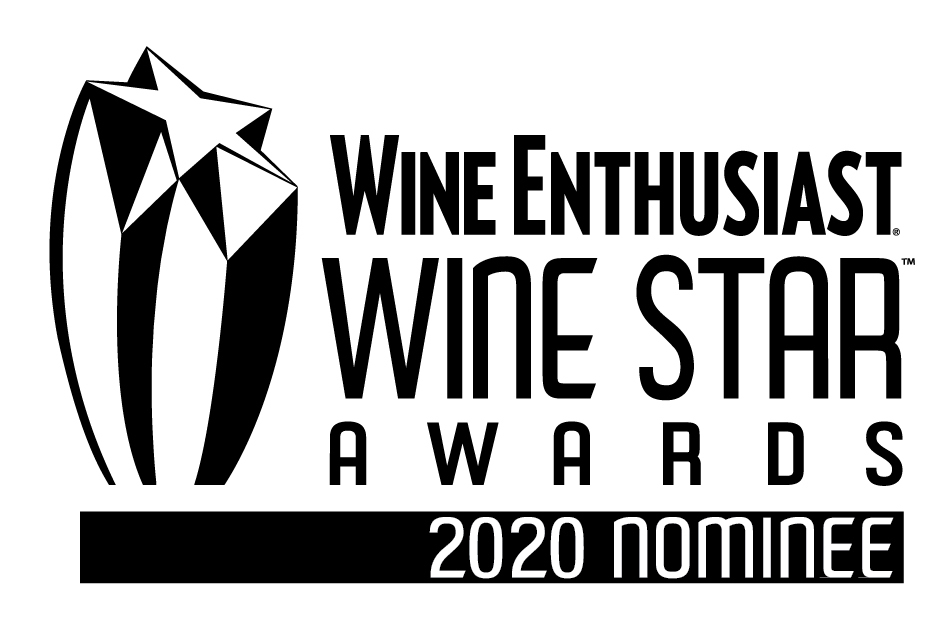The results are in. Congratulations to Cristie Norman who received Wine Enthusiast’s 21st Annual Wine Star Award as 2020's Wine Educator of the Year!

A big hug and thank you to everyone who congratulated me on my nomination. I'm overjoyed to have been considered along with nominees across 15 categories for this year's Wine Star Awards. It's an achievement, no less!

Either way, this is a big win for women industry professionals who have been working towards making the wine space an inclusive space. What an honor to be nominated alongside Cristie and other incredible movers, shakers and disruptors in the wine world. Congratulations to all nominees!

## DISCOUNT OFFER FOR YOU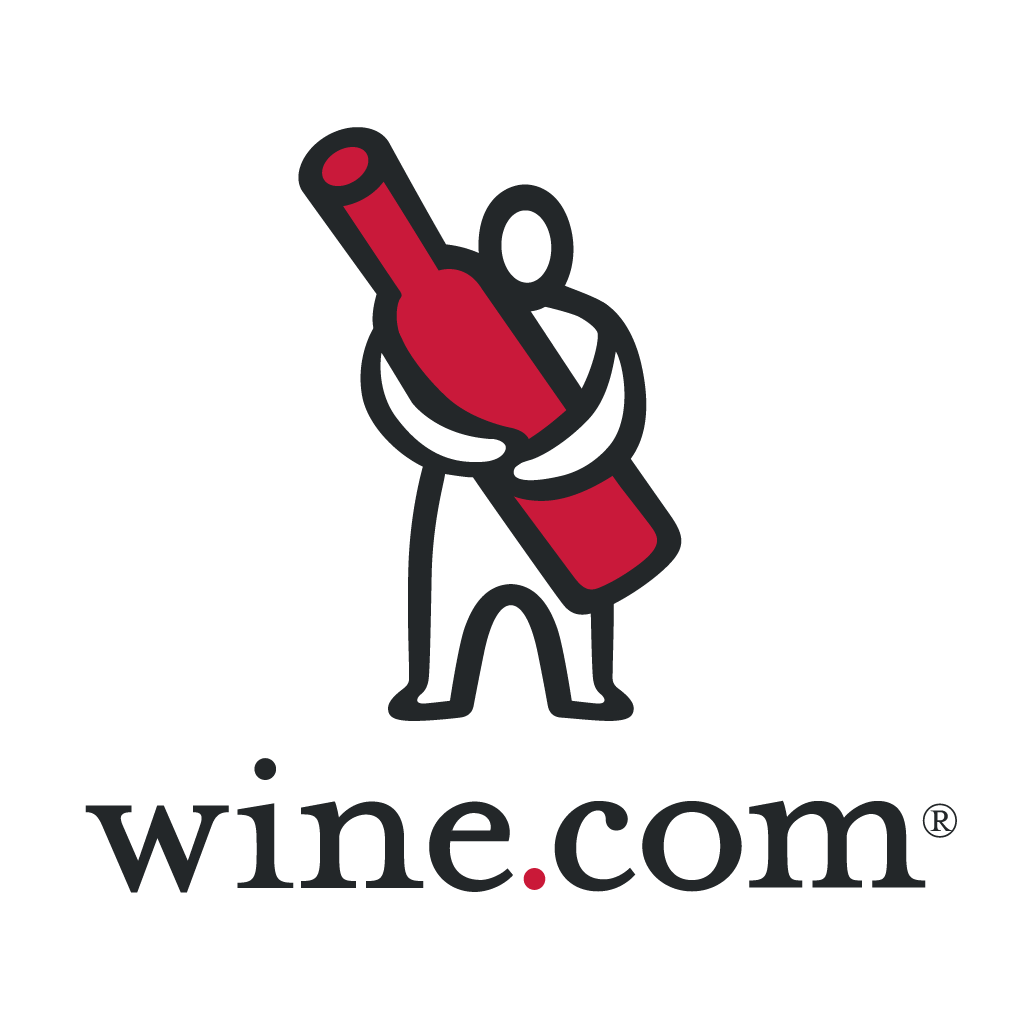Who doesn't love a discount?!

If you're trying to avoid crowds during this time but still want wine, I have a way for you to save some money. How's this for size?

Get \$30 off your first order with Wine.com.

 table div table+table+table+table+table+table+table+table+table+table+table+table+table+table+table+table+table+table+table+table+table+table+table+table+table+table+table+table+table+table+table+table+table+table+table+table+table+table+table+table+table+table+table+table div table{width:100%;padding:0}table div table+table+table+table+table+table+table+table+table+table+table+table+table+table+table+table+table+table+table+table+table+table+table+table+table+table+table+table+table+table+table+table+table+table+table+table+table+table+table+table+table+table+table+table div table img{width:96.23%;padding:0;float:none}table div table+table+table+table+table+table+table+table+table+table+table+table+table+table+table+table+table+table+table+table+table+table+table+table+table+table+table+table+table+table+table+table+table+table+table+table+table+table+table+table+table+table+table+table div table td{width:100%;padding:0 1.88% 18px}/* styles */## BLOG CENTRALWe have a blog! Here you will find things like our Wine of the Week and other special wine & spirits topics! Stay in the know and read up on some of the cool things we've been sharing.

 table div table+table+table+table+table+table+table+table+table+table+table+table+table+table+table+table+table+table+table+table+table+table+table+table+table+table+table+table+table+table+table+table+table+table+table+table+table+table+table+table+table+table+table+table+table+table+table div table{width:100%;padding:0}table div table+table+table+table+table+table+table+table+table+table+table+table+table+table+table+table+table+table+table+table+table+table+table+table+table+table+table+table+table+table+table+table+table+table+table+table+table+table+table+table+table+table+table+table+table+table+table div table img{width:96.23%;padding:0;float:none}table div table+table+table+table+table+table+table+table+table+table+table+table+table+table+table+table+table+table+table+table+table+table+table+table+table+table+table+table+table+table+table+table+table+table+table+table+table+table+table+table+table+table+table+table+table+table+table div table td{width:100%;padding:0 1.88% 18px}/* styles */## BOOK A HOLIDAY EXPERIENCE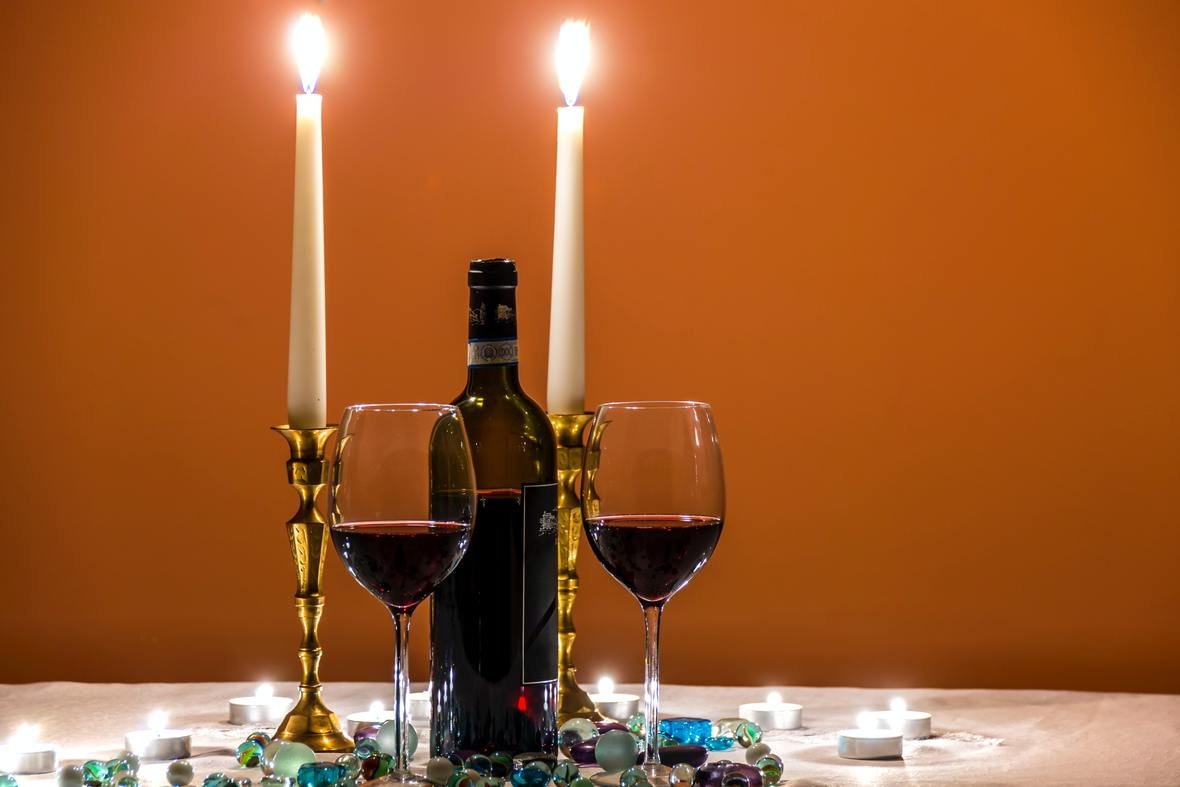Thinking of ways to host a private or corporate holiday party during COVID-19 times?

Or maybe you want to host a cocktail hour for a small group of friends.

Or maybe you want to throw a cocktail hour to celebrate a birthday or special milestone.

Or maybe you're tired of hosting the same kind of virtual events for your team and are looking for something to make them different and engage them.

Whatever the case is, you've come to the right place. Just as Shall We Wine does in-person experiences, we also plan experiences for corporate virtual events. We work with our corporate clients to create wine and spirit-focused experiences!

 table div table+table+table+table+table+table+table+table+table+table+table+table+table+table+table+table+table+table+table+table+table+table+table+table+table+table+table+table+table+table+table+table+table+table+table+table+table+table+table+table+table+table+table+table+table+table+table+table+table+table+table div table{width:100%;padding:0}table div table+table+table+table+table+table+table+table+table+table+table+table+table+table+table+table+table+table+table+table+table+table+table+table+table+table+table+table+table+table+table+table+table+table+table+table+table+table+table+table+table+table+table+table+table+table+table+table+table+table+table div table img{width:96.23%;padding:0;float:none}table div table+table+table+table+table+table+table+table+table+table+table+table+table+table+table+table+table+table+table+table+table+table+table+table+table+table+table+table+table+table+table+table+table+table+table+table+table+table+table+table+table+table+table+table+table+table+table+table+table+table+table div table td{width:100%;padding:0 1.88% 18px}/* styles */## GIVING THANKS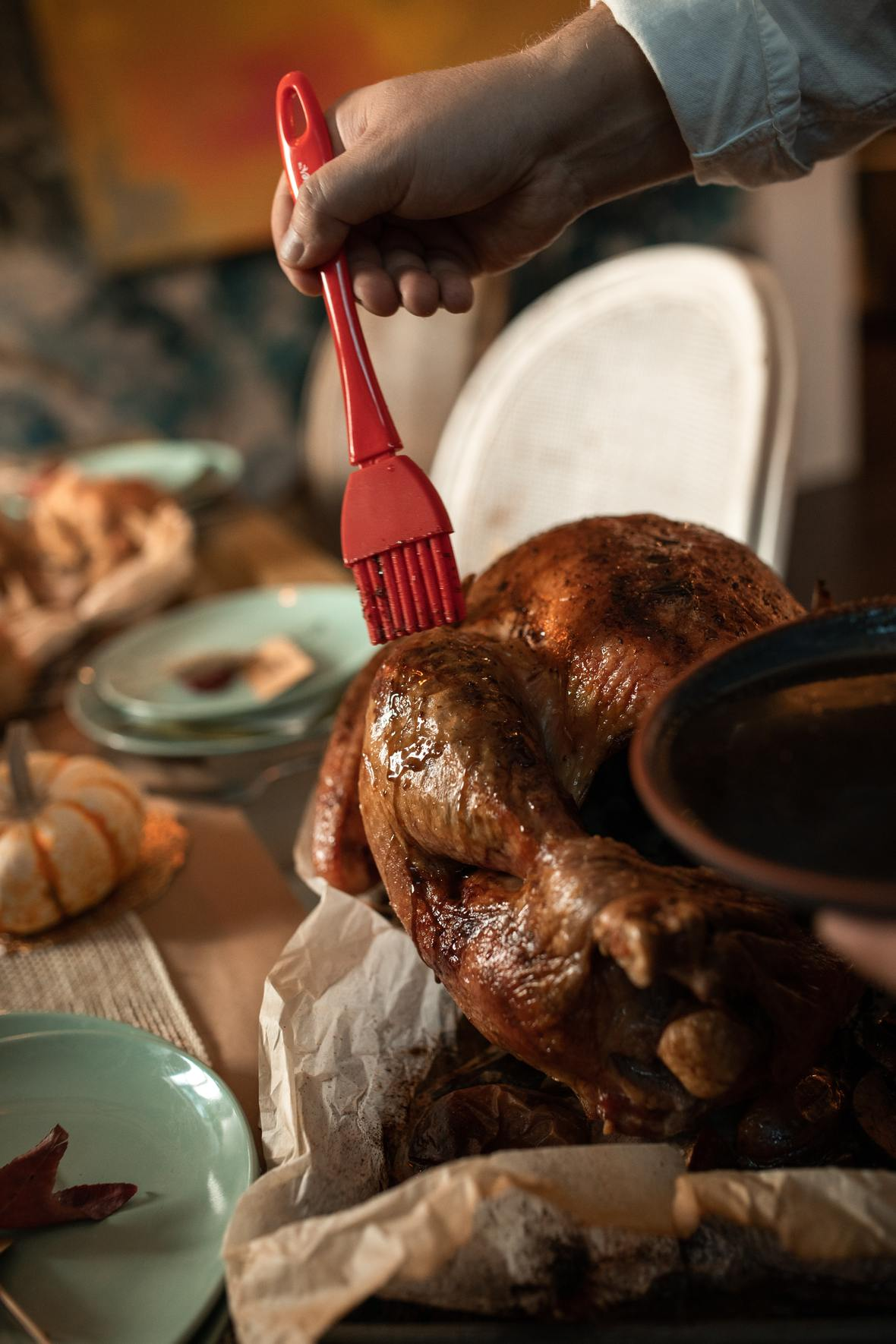During this season of thanks, we would like to thank you, our Shall We Wine tribe.

In a year of numerous unknowns, thank you for supporting this small business. Your support has allowed us to do everything from host interactive virtual wine & spirits events to create educational resources for the budding wine enthusiast. This is love and gratitude from our Shall We Wine family to yours this holiday season.

In an effort to maintain social distancing measures, Shall We Wine will continue to devote our time to connecting with each and every one of our friends and supporters like you virtually. We're constantly sharing fun content to help brighten up your weeks!

We appreciate your patience and cooperation as we work through these times together. Thank you for your continued support in making Shall We Wine your go-to wine & lifestyle resource!/* styles */ Learn, Drink, Repeat! The Shall We Wine Team
 Like   Tweet   in